TEL : +81 48 254 1744

"Easy" Gear Selection Screen

- Choose a gear by pitch, # of teeth, or bore size.
- 2D/3D Drawings Available
- PDF Catalog Available
- Strength Calculation Available

Go to Gear Selection Screen

CLOSE

# Gear Terminology

## 1-3 Gear Terminology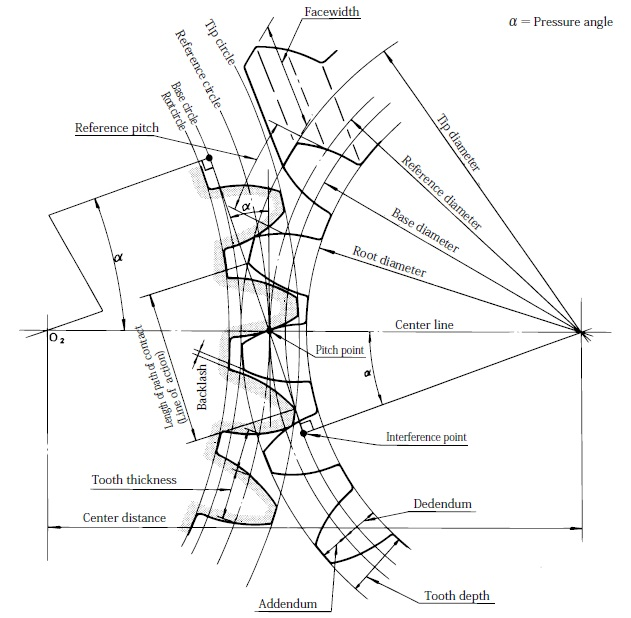Important Gear Terminology in this picture :

• Center distance
• Length of path of contact (Line of action)
• Backlash
• Interference point
• Pitch point
• Reference pitch
• Center line
• Tip circle
• Base circle
• Root circle
• Tip diameter
• Reference diameter
• Base diameter
• Root diameter
• Pressure angle
• Face width
• Tooth depth
• Dedendum
• Tooth thickness

### Gear Module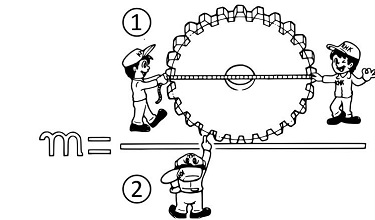1. Reference diameter
2. The number of teeth

Module” is the unit of size that indicates how big or small a gear is. It is the ratio of the reference diameter of the gear divided by the number of teeth. Thus m = d/z (Module = Reference diameter/Number of teeth)

The mutual relation between the module and the reference diameter, etc. is as follows :
Reference diameter d = mz (Reference diameter = Module × Number of teeth)
Number of teeth z = d/m (Number of teeth = Reference diameter / Module)
Reference pitch p = πm (Reference pitch = π x module)

Then, what is the reference pitch ?
It is equal to the circumference divided by the number of teeth.
Reference pitch = Circumference (πd) / Number of teeth (z)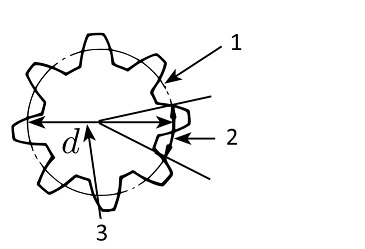1. Circumference
2. Reference pitch
3. Diameter

Then, what is the reference circle ?
Assume that there are two friction pulleys in contact whose diameters are equal to the reference diameters. As the surfaces are smooth, the rotation will not go properly when great force is applied. This problem will be solved if there are teeth on the periphery of the friction pulley. And this is the concept of gearing.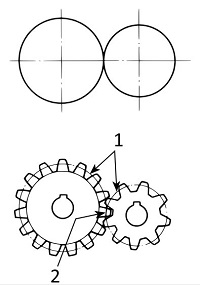1. Friction pulley (Reference circle)
2. Both reference circles come in contact

Summary
(1) The module describes the size of a gear.
(2) A pair of gears can only mesh correctly if and when the base pitch is the same.

### Practicing What You’ve Learned

Spur Gear

Module m = 3
Pinion z1 = 15
Gear z2 = 55

1. Reference diameter
Number of teeth x Module =
z1… ? x ? = ??
z2… ? x ? = ??
2. Tip diameter
Reference diameter + 2 x module =
z1… ? +2 x ? = ??
z2… ? + 2 x ? = ??
3. Center distance
Add reference diameters then divide by two… ? + ? / ? = ??

Helical Gear

Module m = 3
Pinion z1 = 15
Gear z2 = 55
Helix angle β = 16º15′ / then cosβ = 0.96

1. Reference diameter
z1… ? x ? / ? = ??
z2… ? x ? / ? = ??
2. Tip diameter
z1… ? + 2 x ? = ??
z2… ? + 2 x ? = ??
3. Center distance… ? + ? / ? = ??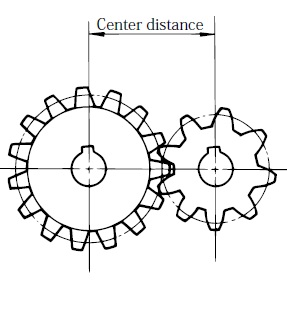Go to Top